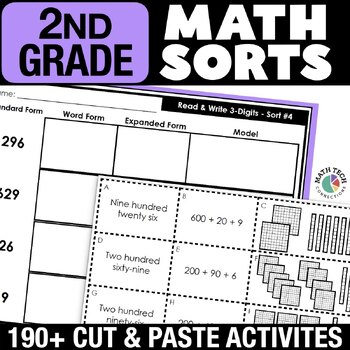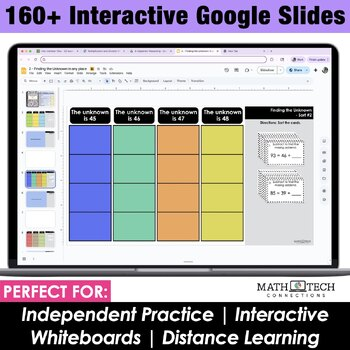# 2nd Grade Math Sorts | 2nd Grade Math Games | Math Interactive Notebook1st - 2nd, Homeschool
Subjects
Standards
Resource Type
Formats Included
• Zip
Pages
234 + Binder
\$25.00
Bundle
List Price:
\$39.00
You Save:
\$14.00
\$25.00
Bundle
List Price:
\$39.00
You Save:
\$14.00

#### Also included in

1. This second grade math bundle includes 3 AMAZING MATH RESOURCES! Included: Math Tri-Folds, Math Sorts, and Math Morning Work!This is a MUST have resource for your 2nd grade classroom. The resources included are flexible and may be used for morning work, whole group practice, guided math practice, in
\$67.50
\$114.00
Save \$46.50

### Description

These math centers are engaging activities that encourage math talk. Students explain how they sort their cards and build strong math concepts as they create cards of their own.

Ways to use: whole group lesson, small group lesson, independent math center, assessment, or homework!

* ALL 2nd Grade MATH Standards Covered *

- Set 1: Operations & Algebraic Thinking - 12 sorts

- Set 2: Number & Operations in Base 10 - 27 sorts

- Set 3: Measurement & Data - 30 sorts

- Set 4: Geometry - 9 sorts

INCLUDED:

- Math Sorts Binder - Pages that list all math sorts, standard, topic, and small group lesson plan page for notes

- Blank PowerPoint Template - Just add text boxes to the template and create your own sorts!

- Student Notebook Dividers - Students keep their math sorts notebook organized with these dividers. Includes learning goals! The dividers are now editable.

There are 2 versions for each sort, so you can differentiate as needed. Version 1 is more challenging, because the category names are not given. Version 2 gives the category names.

You may also be interested in....

MORE MATH SORTS

MATH TRI-FOLDS

Total Pages
234 + Binder
N/A
Teaching Duration
N/A
Report this Resource to TpT
Reported resources will be reviewed by our team. Report this resource to let us know if this resource violates TpT’s content guidelines.

### Standards

to see state-specific standards (only available in the US).
Partition circles and rectangles into two, three, or four equal shares, describe the shares using the words halves, thirds, half of, a third of, etc., and describe the whole as two halves, three thirds, four fourths. Recognize that equal shares of identical wholes need not have the same shape.
Partition a rectangle into rows and columns of same-size squares and count to find the total number of them.
Recognize and draw shapes having specified attributes, such as a given number of angles or a given number of equal faces. Identify triangles, quadrilaterals, pentagons, hexagons, and cubes.
Use addition to find the total number of objects arranged in rectangular arrays with up to 5 rows and up to 5 columns; write an equation to express the total as a sum of equal addends.
Determine whether a group of objects (up to 20) has an odd or even number of members, e.g., by pairing objects or counting them by 2s; write an equation to express an even number as a sum of two equal addends.